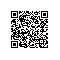# PHP：php知识小解

PHP 是一种创建动态交互性站点的服务器端脚本语言。
PHP 能够生成动态页面内容。
PHP 能够创建、打开、读取、写入、删除以及关闭服务器上的文件。
PHP 能够接收表单数据并处理。
PHP 能够添加、删除、修改数据库中的数据。
PHP 能够限制用户访问网站中的某些页面。
PHP 能够对数据进行加密和解密。
....

Java.
net...PHP格式：

PHP 脚本以<?php 开头，以 ?> 结尾，其文件的默认文件扩展名是.php，其语句以分号(;)结尾。

php脚本的后面名为.php，代码放置在下面的括号里面：

<?php
.......
?>

echo可以打印信息，类似于printf

<?php
echo "hallo world";
?>

php支持三种注释方式：

<!DOCTYPE html>
<html>
<body>
<?php
ECHO "Hello World!<br>";
echo "Hello World!<br>";
Echo "Hello World!<br>";
?>
</body>
</html>

<?php
$color="red";$Color="black";
echo "my car is " . $color . "<br>"; echo "my car is " .$Color . "<br>";
?>

PHP 变量规则：

function myTest() {
$y=10; // 局部作用域 echo "<p>测试函数内部的变量：</p>"; echo "变量 x 是：$x";
echo "<br>";
echo "变量 y 是：$y"; } myTest(); echo "<p>测试函数之外的变量：</p>"; echo "变量 x 是：$x";
echo "<br>";
echo "变量 y 是：$y"; ?> 运行结果： 测试函数内部的变量： 变量 x 是： 变量 y 是：10 测试函数之外的变量： 变量 x 是：5 变量 y 是： 比较奇怪的就是为什么全局变量不能在局部函数内访问。 其实可以访问，不过需要global关键字的帮助： global 关键词用于访问函数内的全局变量。 要做到这一点，请在（函数内部）变量前面使用 global 关键词： <?php$x=5;
$y=10; function myTest() { global$x,$y;$y=$x+$y;
}

myTest();
echo $y; // 输出 15 ?> PHP 同时在名为$GLOBALS[index] 的数组中存储了所有的全局变量。下标存有变量名。这个数组在函数内也可以访问，并能够用于直接更新全局变量。

<?php
$x=5;$y=10;

function myTest() {
$GLOBALS['y']=$GLOBALS['x']+$GLOBALS['y']; } myTest(); echo$y; // 输出 15
?>

<?php

function myTest() {
static $x=0; echo$x;
$x++; } myTest(); myTest(); myTest(); ?> php中echo和print都能使用，两者的唯一区别是print返回1，echo没有返回值。 strlen函数可以返回字符串的长度。 strpos函数用来确定另外一个字符串的位置： <?php echo strpos("Hello world!","world"); ?> 完整的string参考手册. 使用define函数来定义常量： <?php define("GREETING", "Welcome to W3School.com.cn!"); echo GREETING; ?> define函数还有第三个参数，用来指定是否大小写敏感。 php的if-else语句和其他语言大同小异，举个例子： <?php$t=date("H");

if ($t<"10") { echo "Have a good morning!"; } elseif ($t<"20") {
echo "Have a good day!";
} else {
echo "Have a good night!";
}
?>

switch-case语句：

<?php
switch ($x) { case 1: echo "Number 1"; break; case 2: echo "Number 2"; break; case 3: echo "Number 3"; break; default: echo "No number between 1 and 3"; } ?> while、for语句和其他语言无差别，看看foreach吧： <?php$colors = array("red","green","blue","yellow");

foreach ($colors as$value) {
echo "$value <br>"; } ?> php的真正力量来自于它的函数，它有1000个内置函数。 用户定义的函数声明以关单 "function" 开头： function functionName() { 被执行的代码; } 举个例子： <?php function writeMsg() { echo "Hello world!"; } writeMsg(); // 调用函数 ?> // 含参数 <?php function familyName($fname,$year) { echo "$fname Zhang. Born in $year <br>"; } familyName("Li","1975"); familyName("Hong","1978"); familyName("Tao","1983"); ?> // 默认参数 <?php function setHeight($minheight=50) {
echo "The height is : $minheight <br>"; } setHeight(350); setHeight(); // 将使用默认值 50 setHeight(135); setHeight(80); ?> // 返回值 <?php function sum($x,$y) {$z=$x+$y;
return $z; } echo "5 + 10 = " . sum(5,10) . "<br>"; echo "7 + 13 = " . sum(7,13) . "<br>"; echo "2 + 4 = " . sum(2,4); ?> 在 PHP 中，有三种数组类型： • 索引数组 - 带有数字索引的数组 • 关联数组 - 带有指定键的数组 • 多维数组 - 包含一个或多个数组的数组 索引数组： $cars=array("Volvo","BMW","SAAB");
<?php
$cars=array("Volvo","BMW","SAAB"); echo "I like " .$cars . ", " . $cars . " and " .$cars . ".";
?>

// count
<?php
$cars=array("Volvo","BMW","SAAB"); echo count($cars);
?>

// 变量索引数组
<?php
$cars=array("Volvo","BMW","SAAB");$arrlength=count($cars); for($x=0;$x<$arrlength;$x++) { echo$cars[$x]; echo "<br>"; } ?> 关联数组： $age=array("Peter"=>"35","Ben"=>"37","Joe"=>"43");

$age['Peter']="35";$age['Ben']="37";
$age['Joe']="43"; <?php$age=array("Bill"=>"35","Steve"=>"37","Peter"=>"43");
echo "Peter is " . $age['Peter'] . " years old."; ?> // 遍历 <?php$age=array("Bill"=>"35","Steve"=>"37","Peter"=>"43");

foreach($age as$x=>$x_value) { echo "Key=" .$x . ", Value=" . $x_value; echo "<br>"; } ?> 数组排序方法有下面这些： sort() - 以升序对数组排序 rsort() - 以降序对数组排序 asort() - 根据值，以升序对关联数组进行排序 ksort() - 根据键，以升序对关联数组进行排序 arsort() - 根据值，以降序对关联数组进行排序 krsort() - 根据键，以降序对关联数组进行排序 比较难理解的是键值对排序。 <?php$age=array("Bill"=>"35","Steve"=>"37","Peter"=>"43");
asort($age); ?> <?php$age=array("Bill"=>"35","Steve"=>"37","Peter"=>"43");
ksort($age); ?> 超全局变量，也就是预定义的全局变量，在哪里都能用，有特殊含义： $GLOBALS:引用全局作用域中可用的全部变量
$_SERVER:保存关于报头、路径和脚本位置的信息。$_REQUEST:用于收集 HTML 表单提交的数据。
$_POST:用于收集提交 method="post" 的 HTML 表单后的表单数据。也常用于传递变量。$_GET:$_GET 也可用于收集提交 HTML 表单 (method="get") 之后的表单数据。$_FILES
$_ENV$_COOKIE
\$_SESSION使用钉钉扫一扫加入圈子
+ 订阅